CAT  >  Test: Logarithm- 2

# Test: Logarithm- 2

Test Description

## 10 Questions MCQ Test General Aptitude for GATE | Test: Logarithm- 2

Test: Logarithm- 2 for CAT 2023 is part of General Aptitude for GATE preparation. The Test: Logarithm- 2 questions and answers have been prepared according to the CAT exam syllabus.The Test: Logarithm- 2 MCQs are made for CAT 2023 Exam. Find important definitions, questions, notes, meanings, examples, exercises, MCQs and online tests for Test: Logarithm- 2 below.
Solutions of Test: Logarithm- 2 questions in English are available as part of our General Aptitude for GATE for CAT & Test: Logarithm- 2 solutions in Hindi for General Aptitude for GATE course. Download more important topics, notes, lectures and mock test series for CAT Exam by signing up for free. Attempt Test: Logarithm- 2 | 10 questions in 10 minutes | Mock test for CAT preparation | Free important questions MCQ to study General Aptitude for GATE for CAT Exam | Download free PDF with solutions
 1 Crore+ students have signed up on EduRev. Have you?
Test: Logarithm- 2 - Question 1

### Which of the following statements is not correct?

Detailed Solution for Test: Logarithm- 2 - Question 1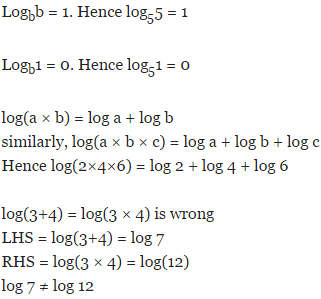Test: Logarithm- 2 - Question 2

### If log 2 = 0.3010 and log 3 = 0.4771, the value of log5 512 is:

Test: Logarithm- 2 - Question 3

###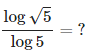Detailed Solution for Test: Logarithm- 2 - Question 3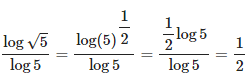Test: Logarithm- 2 - Question 4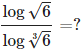Detailed Solution for Test: Logarithm- 2 - Question 4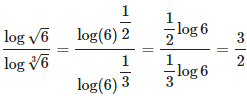Test: Logarithm- 2 - Question 5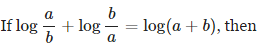Detailed Solution for Test: Logarithm- 2 - Question 5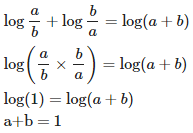Test: Logarithm- 2 - Question 6

If log(64)= 1.806, log(16) = ?

Detailed Solution for Test: Logarithm- 2 - Question 6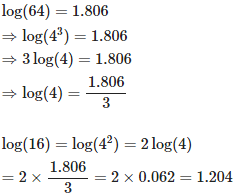Test: Logarithm- 2 - Question 7

If log 2 = 0.3010 and log 3 = 0.4771, What is the value of log51024?

Detailed Solution for Test: Logarithm- 2 - Question 7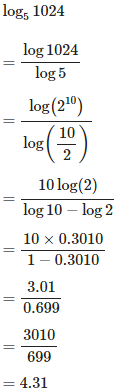Test: Logarithm- 2 - Question 8

if log 2 = 0.30103 and log 3 = 0.4771, find the number of digits in (648)5.

Detailed Solution for Test: Logarithm- 2 - Question 8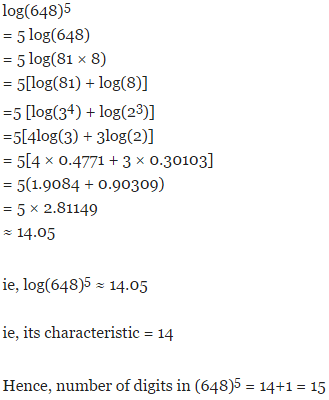Test: Logarithm- 2 - Question 9

if log 2 = 0.30103, the number of digits in 2128 is

Detailed Solution for Test: Logarithm- 2 - Question 9

log(2128) = 128log(2) = 128 × 0.30103 ≈ 38.4

ie, its characteristic = 38

Hence, number of digits in 2128 = 38+1 = 39

Test: Logarithm- 2 - Question 10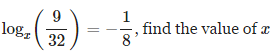Detailed Solution for Test: Logarithm- 2 - Question 10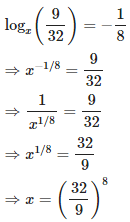## General Aptitude for GATE

73 videos|86 docs|108 tests
Information about Test: Logarithm- 2 Page
In this test you can find the Exam questions for Test: Logarithm- 2 solved & explained in the simplest way possible. Besides giving Questions and answers for Test: Logarithm- 2, EduRev gives you an ample number of Online tests for practice

## General Aptitude for GATE

73 videos|86 docs|108 tests

### How to Prepare for CAT

Read our guide to prepare for CAT which is created by Toppers & the best Teachers(Scan QR code)